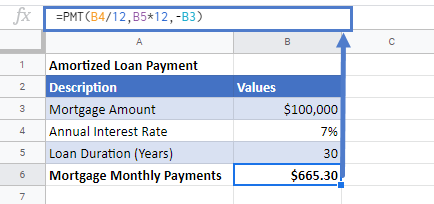# Calculate Loan Payments in Excel & Google Sheets

In this tutorial, we will learn how to calculate the monthly payments for any type of loan in excel & google sheets.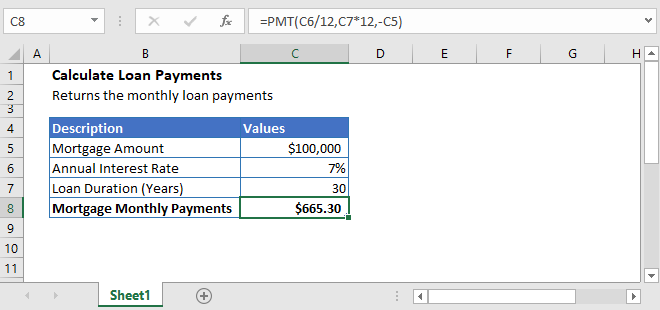## Calculate Loan Payments

Calculating loan payments is easy, whether it’s for mortgages, cars, students, or credit cards. It’s important to know what type of loan you’re getting, either it’s an interest-only loan or amortizing loan. Because the methods to calculate loan payments are different.

The loan types and how to calculate loan payments for it are explained below.

## Interest-only Loan Payment

An interest-only loan is the one in which the borrower pays only the interest for a certain period of time.

These types of loan’s monthly payments can be calculated by multiplying the interest rate of the loan with the loan amount and dividing it by 12.

### Interest-only Mortgage Payment Calculation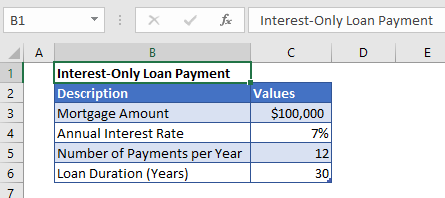For example, let’s calculate the monthly payments of an interest-only mortgage. If the interest rate on a 30-year mortgage is 7% and the amount of mortgage is \$100,000.

The monthly payments fo the mortgage are calculated by putting the interest-only loan payment formula in cell C7: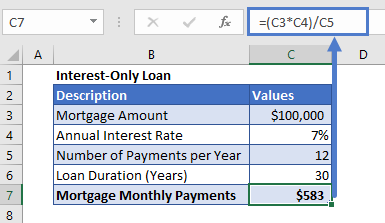## Amortized Loan Payment

An amortized loan is a type of loan for which the loan amount plus the interest owed is paid off over a set period of regular payments.

The general formula to calculate payment from this type of loan is

The above formula is kind of a complex one.

Thankfully, Excel has made it easy for you to calculate loan payments for any type of loan or credit card. Excel has a built-in function, PMT, that calculates the monthly loan payments for you. All you have to do is enter the details of the loan like the interest rate, the duration, and the principal of the loan and Excel will calculate the loan payments for you.

The syntax for the PMT function is:

### Calculate Amortized Mortgage Monthly Payments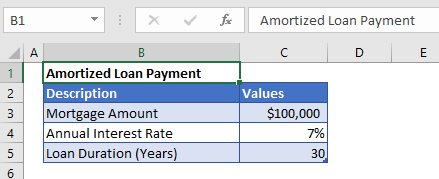Let’s take the previous example and calculate the amortized loan payment for it.

Before applying the PMT function, we need to make sure that the interest rate and the payment period’s units are consistent.

And to do that, the annual interest rate is converted into monthly interest rate by dividing it with 12 and similarly, the payment periods are also converted into monthly payment periods by multiplying its value with 12. Also, the mortgage loan payment is entered with a negative sign in the formula,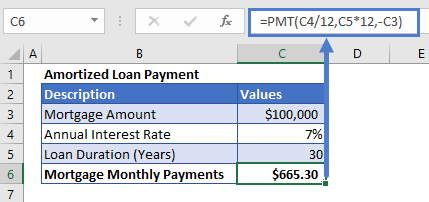Here, we haven’t entered the fv and type arguments’ values because we don’t need them.

## Calculate the loan payments in Google Sheets

The formula to calculate the loan payments works exactly the same in Google Sheets as in Excel: